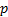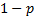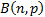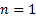/

### The Bernoulli distribution

A Bernoulli trial is an experiment that has one of two possible outcomes, ‘success’ with probabilityand ‘failure’ with probability. The Bernoulli distribution is a probability distribution that takes the value of 1 if such a trial is a ‘success’ and 0 if it is a ‘failure’.

The Bernoulli distribution is a special case of the binomial distribution,, with. For characteristics of the Bernoulli distribution (e.g. mean, standard deviation etc.), please refer to the corresponding characteristics for the binomial distribution.

For other probability distributions see here.